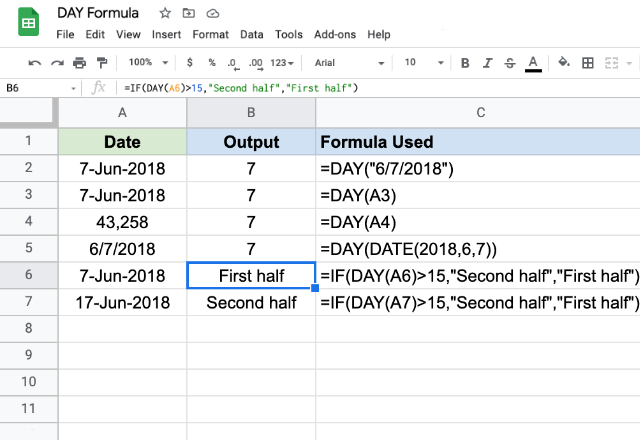# How to use the DAY formula in Google SheetsThere can be situations where we’d like to extract the day of the month from a given date. Probably to use it with another formula that decides if it falls in the first half or second half of the month. On a quick look, we can quickly identify the day of the month. But the machine doesn’t know it directly. Because a date is just a numeric value for any spreadsheets application. For example, the underlying numeric value for the date “7-Jun-2018” is 43,258. So how do we extract the day of the month from this numeric value? The DAY formula is available for just this purpose, to calculate the Google Sheets day of month formula.

### Syntax

DAY(date)

date – is the date which we need to extract the day from. This input has to be a date type data. We can ensure that either by using formulas such as DATE, DATEVALUE or TO_DATE that return date objects. Or we may also use references to the cells that have dates or numbers.

### Usage: Google Sheets day of month formula

The syntax we just learned suggests that the DAY formula in Google Sheets is pretty simple and straightforward. Let us further reinforce our understanding with the help of a few practical examples.As you may have noticed we have tried various ways in which we can pass the input parameter value. In the first example, we passed a valid date string, and the formula, as expected returned 7. The second example demonstrates the capability of the formula to accept the references to cells that have dates within them. The third example worked even when there’s a number on the cell. Co-incidentally the number 43,258 is equivalent to the date 7-Jun-2017 in Google Sheets. Hence it returned the value 7. On the fourth example, we tried embedding a DATE function within the formula.

While the first four examples demonstrate the working of the formula itself, we tried more practical examples on the rows 6 and 7. Here we are trying to deduce whether a day belongs to the first half of the month or the second. And the composite formula delivers its job religiously indicating which day belongs to which half.